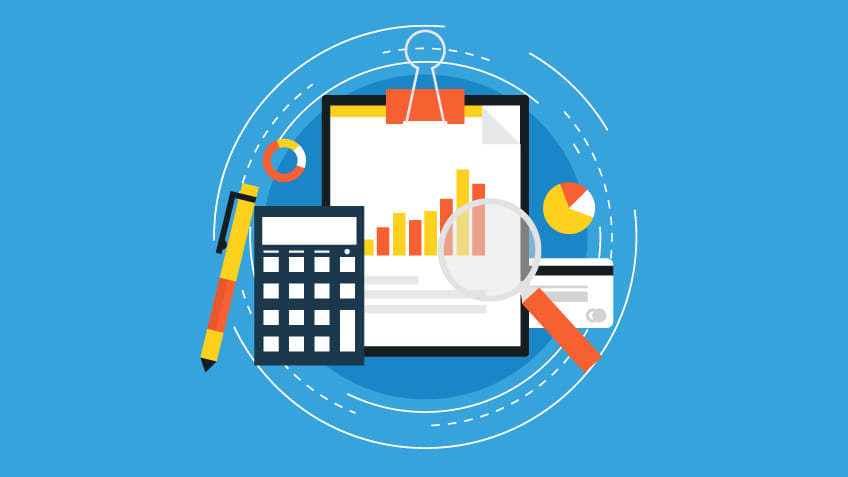The PMBOK® Guide clearly states how to calculate Estimate at Completion (EAC) in different situations. I can tell you from my personal experience that the EAC calculation questions you may get during your PMP® examination can be quite confusing. The PMP® exam assesses your ability to understand the concept of EAC as well as the correct application of EAC in each project—so you need to understand the different ways of calculating EAC.

## EAC Calculation Categories

There are four general categories for EAC calculation.

They are as follows:

1. EAC = AC + Bottom-up ETC

This formula is used when the original estimation is fundamentally flawed. It calculates the actual plus new estimate for the remaining work.

2. EAC =BAC/Cumulative CPI

This formula is used when the original estimation is met without any deviation. It signifies that your project is going well: you are maintaining the CPI and SPI as 1, and you should continue the project in the same way. It is always good for a project manager if he or she is maintaining the CPI and SPI as 1 or even more than 1.

3. EAC = AC + (BAC - EV)

This formula is used when the current deviation with the original estimation is thought to be different in the future. It is generally AC plus the remaining value of the work to perform.

4. EAC = AC + [BAC - EV / (Cumulative CPI x Cumulative SPI)]

This formula is used to calculate actual to date plus the remaining budget changed based on the performance. It is used when we believe the current ratio is typical as planned. In other words, we have to meet the schedule earlier than originally determined, and we calculate the EAC accordingly to meet that schedule.### Example 1:

Abraham is the project manager for an ITES project. Suddenly, there is a scope change request from the customer, and upper management asked for a new estimate of the total cost of the project with this new implemented scope on the project. The project has already incurred an amount of \$250,000 and has a CPI of 1.08. The project manager discussed with the team and all the stakeholders and decided on some future investments, such as admin costs of \$60,000, quality control costs at \$25,000, and miscellaneous costs as \$11,000. What is the estimate at completion in this case?

#### How do you calculate this?

In this example, the original estimates are poor because they are based on a flawed approach. Therefore, you should calculate EAC using the formula from condition 1 here: Estimate at Completion = Actual Cost + Bottom-up Estimate to Complete By using the abovementioned formula, you can calculate: \$250,000 + (\$60,000 + \$25,000 + \$11,000) = \$346,000.

### Example 2:

Kate is a project manager who is managing a large and complex project. Midway through the project, upper management asked her for an updated estimate of the total cost of the project. At the beginning of the project, the costs of the project were estimated at \$150,000 for development, \$170,000 for design costs, and \$120,000 for quality control. The Cost Performance Index of the project is 1.04. What is the Estimate at Completion at this stage?

#### How do you calculate this?

In this example, the CPI is not considered abnormal, so the formula using CPI (condition 2) will be in use:

Estimate at Completion = Budget at Completion / Cost Performance Index

By using the above-mentioned formula, you can calculate: (\$150,000 + \$170,000 + \$120,000) / 1.04 = \$423,076.92.

### Example 3:

Dave is the project manager for a software company. He and his team are working on a project to develop software for their customer. During execution, the project manager realized that mistakes were made while collecting the project requirements. Dave has fixed the mistakes and put a mitigation plan in place. At the start of the project, the costs of the project were estimated at \$200,000 for design, \$300,000 for development, and \$200,000 for quality control. The project has spent \$400,000 so far. The value of the work completed is \$500,000. What is the Estimate at Completion?

#### How do you calculate this?

In this example, the CPI is considered abnormal. So you can calculate Estimate at Completion using the formula from condition 3:

Estimate at Completion = Actual Cost + (Budget at Completion – Earned Value)

By using the above-mentioned formula: \$400,000 + (\$700,000 – \$500,000) = \$600,000

### Example 4:

Lisa is working on a project. The CEO has told the shareholders that the new system will be in place in six months, without discussing this first with the PMO. At the start of the project, the costs of the project were estimated at \$150,000 for design, \$700,000 for development, and \$225,000 for quality assurance. The project has spent \$450,000 so far. The CPI for the project is 0.9, and the SPI is 0.8. The value of the work completed is \$375,000. What is the Estimate at Completion?

#### How do you calculate?

In this example, the CPI is considered abnormal. So you can calculate Estimate at Completion using the formula from condition 4:
Estimate at Completion = Actual Cost + [(Budget at Completion - Earned Value) / (Cost Performance Index x Schedule Performance Index)]
By using the above-mentioned formula: = \$450,000 + [(\$1,075,000 - \$375,000) / (0.9 x 0.8)] = \$450,000 + [\$700,000 / 0.72] = \$450,000 + \$972,222.23 = \$1,422,222.23

 Enroll in our PMP® Certification Course today and develop a strong foundation in the principles of project management.

## Conclusion

Now that you have a better understanding of the different ways to calculate the Estimate at Completion, you’re well on your way to attaining the PMP® certification. Want more project management training? Simplilearn offers everything you need to train for your PMP® exam and pass it on the first try.

PMBOK®, PMP®, and PMI® are registered trademarks of the Project Management Institute, Inc.Simplilearn